# Fundamental Forces in Nature

#### description

• Fundamental Forces of Physics
• Gravitational Force
• Electromagnetic Force
• Strong Nuclear Force
• Weak Nuclear Force
• Towards Unification of Forces

#### notes

Fundamental forces in nature

The Four Fundamental Forces and their strengths

1. Gravitational Force – Weakest force; but infinite range.

2. Weak Nuclear Force – Next weakest; but short range.

3. Electromagnetic Force – Stronger, with infinite range.

4. Strong Nuclear Force – Strongest; but short range.

 Name Relative Strength Range Operates among Gravitational force 10–39 Infinite All objects in the universe Weak nuclear force 10–13 Very short, Sub-nuclear size (10-16m) Some elementary particles, particularly electron and neutrino Electromagnetic force 10–2 Infinite Charged particles Strong nuclear force 1 Short, nuclear size (-10-15m) Nucleons, heavier elementary particles

Gravitational Force:

It is the force of mutual attraction between any two objects by virtue of their masses. It is a universal force as every object experiences this force due to every other object in the universe.

The gravitational force is weak, but very long ranged. Furthermore, it is always attractive. It acts between any two pieces of matter in the Universe since mass is its source.

Gravity is a force in which it pulls anything down to the centre of the Earth. There are unlimited examples of gravitational forces.

• Your weight- If there was no gravity, you would weigh nothing

• Apples falling from a tree -The reason how gravity was discovered (by Issac Newton)

• Kicking a football up in the air; it obviously comes back down

• Jumping up and down

• Rain falling from the sky

• All planets forming the solar system- The reason why planets are orbiting the Sun is because of its huge gravitational pull

• The moon orbiting the Earth-

• Waves in the sea are formed mostly due to the gravity of the moon

• The Milky Way galaxy is spinning due to the enormous gravitational pull of the super-massive black hole in the very centre which is 4 million times the mass of our Sun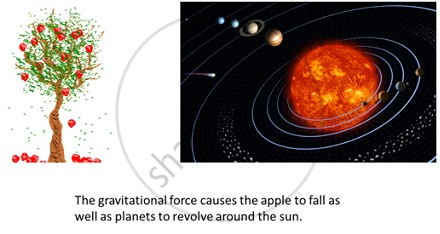Weak Nuclear Force:-

The weak force is responsible for radioactive decay and neutrino interactions. The weak force causes Beta decay. In β- decay, the nucleus emits an electron and an uncharged particle called neutrino. It has a very short range. As its name indicates, it is very weak.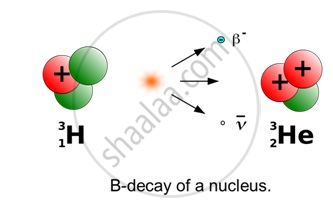Electromagnetic Force:-

It is the force between charged particles. Charges at rest have electric attraction (between unlike charges) and repulsion (between like charges). Charges in motion produce magnetic force. Together they are called Electromagnetic Force.

The electromagnetic force causes electric and magnetic effects such as the repulsion between like electrical charges or the interaction of bar magnets. It is long-ranged, but much weaker than the strong force. It can be attractive or repulsive, and acts only between pieces of matter carrying electrical charge. Electricity, magnetism, and light are all produced by this force.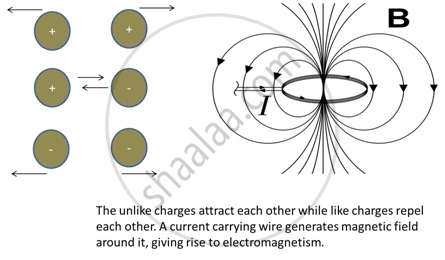Strong Nuclear Force:-

It is the attractive force between protons and neutrons in a nucleus. It is charge-independent and acts equally between a proton and a proton, a neutron and a neutron, and a proton and a neutron. Recent discoveries show that protons and neutrons are built of elementary particles, quarks.

The strong interaction is very strong, but very short-ranged. It is responsible for holding the nuclei of atoms together. It is basically attractive, but can be effectively repulsive in some circumstances. The strong force is carried by particles called gluons; that is, when two particles interact through the strong force, they do so by exchanging gluons. Thus, the quarks inside of the protons and neutrons are bound together by the exchange of the strong nuclear force.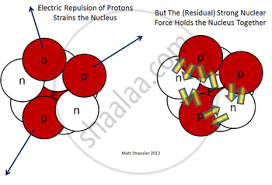Unification of Forces:-

There have been physicists who have tried to combine a few of the above fundamental forces. These are listed in table below.

 Name of Physicist Year Achievement in Unification Isaac Newton 1687 Unified celestial and terrestrial mechanics. Hans Christian Oersted and Michael Faraday 1820 and 1830 respectively Unified electric and magnetic phenomena to give rise to electromagnetism. James Clerk Maxwell 1873 Unified electricity, magnetism and optics to show that light is an electromagnetic wave. Sheldon Glashow, Abdus Salam, Steven Weinberg Carlo Rubia, Simon Vander Meer 1979     1984 Gave the idea of electro-weak force which is a combination of electromagnetic and weak nuclear force.   Verified the theory of electro-weak force.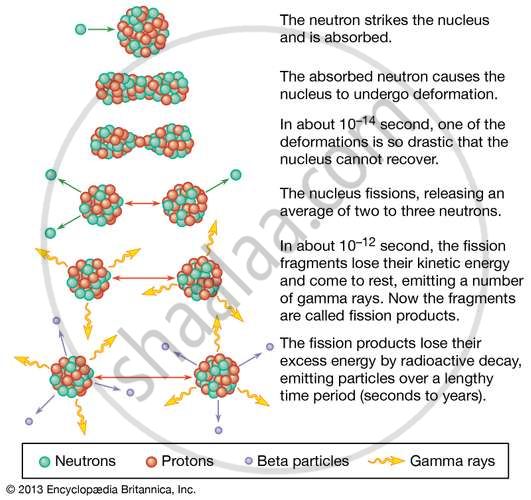If you would like to contribute notes or other learning material, please submit them using the button below.

### Shaalaa.com

Force in Physics [00:06:20]
S
0%